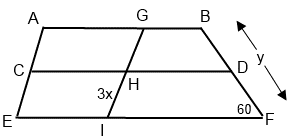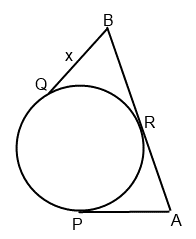+91-22-62437100 / 01 | help@reachivy.com

# GMAT Data Sufficiency Quiz 3

Data Sufficiency test may not have been a part of your academic curriculum, but it is an important aspect of your GMAT. If you are unsure about Data Sufficiency, and your own ability to crack the test, here is a sample test to help you understand the test.

Instructions: This data sufficiency problem consists of a question and two statements, labeled (1) and (2), in which certain data are given. You have to decide whether the data given in the statements are sufficient for answering the question. Using the data given in the statements, plus your knowledge of mathematics and everyday facts (such as the number of days in July or the meaning of the word counterclockwise), you must indicate whether:

Statement (1) ALONE is sufficient, but statement (2) alone is not sufficient to answer the question asked.
Statement (2) ALONE is sufficient, but statement (1) alone is not sufficient to answer the question asked.
BOTH statements (1) and (2) TOGETHER are sufficient to answer the question asked, but NEITHER statement ALONE is sufficient to answer the question asked.
Statements (1) and (2) TOGETHER are NOT sufficient to answer the question asked, and additional data specific to the problem are needed.

Welcome to our GMAT Data Sufficiency Quiz 3. To begin click Next

Question 1:
Line P is parallel to Line Q on the xy-coordinate plane. What is the shortest distance between the two lines?
(1) Line Q has a slope of 2.
(2) Line P crosses the y-axis at 8.

Question 2:

Five circles are inscribed in a larger circle such that one of the five circles is placed exactly in the middle of the larger circle and tangent to the other four circles. The outer four small circles are tangent to the larger circle, but are not tangent to one another. What is the area of the region inside the larger circle but outside the smaller circles?
(1) The diameter of each smaller circle is 6π.
(2) The diameter of the large circle passes through the diameter of three of the smaller circles.

Question 3:

Right triangle ABC has a hypotenuse of 30. Side leg BC = 9x. What is the length of leg AB?
(1) Point D lies on BC such that DC = (4/9)BC.

Question 4:If AC = CE, and all horizontal lines in the figure are parallel, what is the value of y/x?
(1) BD = 20
(2) GH = 18

Question 5:If AB = 14 and AP, BQ, and AB are tangents, what is the value of x?
(1) The radius of the circle is 6.
(2) PA = 6

Question 6:

Three tennis balls fit into a cylindrical container such that the tennis balls touch the bottom and top of the can. If the formula for the volume of sphere is 4/3(πr3), what fraction of the volume of the container is occupied by the tennis balls?
(1) The radius of the container is 6.
(2) The radius of each tennis ball equals the radius of the container.

You have answered all the questions in the quiz. Press Submit to view your score